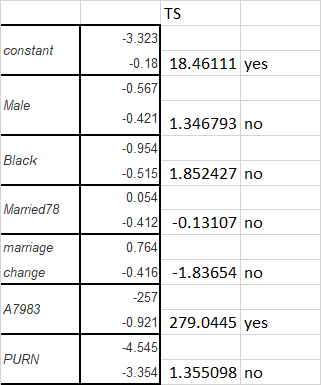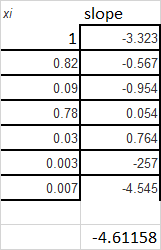##### Question

In: Statistics and Probability

# A study investigated the impact of house price appreciation on household mobility. The underlying idea was...

A study investigated the impact of house price appreciation on household mobility. The underlying idea was that if a house were viewed as one part of the household's portfolio, then changes in the value of the house, relative to other portfolio items, should result in investment decisions altering the current portfolio. Using 5,162 observations, the logit equation was estimated as shown in the table, where the limited dependent variable is one if the household moved in 1978 and is zero if the household did not move:

 Regression model Logit constant -3.323 (0.180) Male -0.567 (0.421) Black -0.954 (0.515) Married78 0.054 (0.412) marriage change 0.764 (0.416) A7983 -0257 (0.921) PURN -4.545 (3.354) Pseudo-R2 0.016

where male, black, married78, and marriage change are binary variables. They indicate, respectively, if the entity was a male-headed household, a black household, was married, and whether a change in marital status occurred between 1977 and 1978. A7983 is the appreciation rate for each house from 1979 to 1983 minus the SMSA-wide rate of appreciation for the same time period, and PNRN is a predicted appreciation rate for the unit minus the national average rate.

(a) Interpret the results. Comment on the statistical significance of the coefficients. Do the slope coefficients lend themselves to easy interpretation?

(b) The mean values for the regressors are as shown in the accompanying table.

 Variable Mean male 0.82 black 0.09 married78 0.78 marriage change 0.03 A7983 0.003 PNRN 0.007

Taking the coefficients at face value and using the sample means, calculate the probability of a household moving.

(c)   Given this probability, what would be the effect of a decrease in the predicted appreciation rate of 20 percent, that is A7983 = –0.20?

## Solutions

##### Expert Solution

a)

TS = b^ / se(b^)sincen is very large, |TS| > 1.96 ,variable is significant

here onlt A7983 is significant

b)

log(p /(1-p)) = b0+ b1 *x1 + b2*x2+..

b0 + b1*x1+ ..log(p/(1-p)) = -4.61158

p = 1/(1+ e^(4.61158))

= 0.0098

c)

slope = -257

it means when it is is reduced by 20% , log odds is increased 257*0.2

= 51.4

## Related Solutions

##### Q2. Identify a recent study in the literature which has investigated the impact of income on...
Q2. Identify a recent study in the literature which has investigated the impact of income on the demand for health care? 2.1. Give the full reference for your chosen study. 2.2. Write a short paragraph (3-4 sentences) to summarize how income is correlated with the demand for health care?
##### Explain how the time to expiration, volatility, and price of the underlying stock impact but put...
Explain how the time to expiration, volatility, and price of the underlying stock impact but put and call options prices.
##### The central issue underlying the study of leverage is whether or not it influences stock price...
The central issue underlying the study of leverage is whether or not it influences stock price and whether there’s an optimal structure. But the whole idea seems kind of fuzzy and uncertain. Why are people so interested? (Hint: Think of management’s goals and of the world of mergers.)